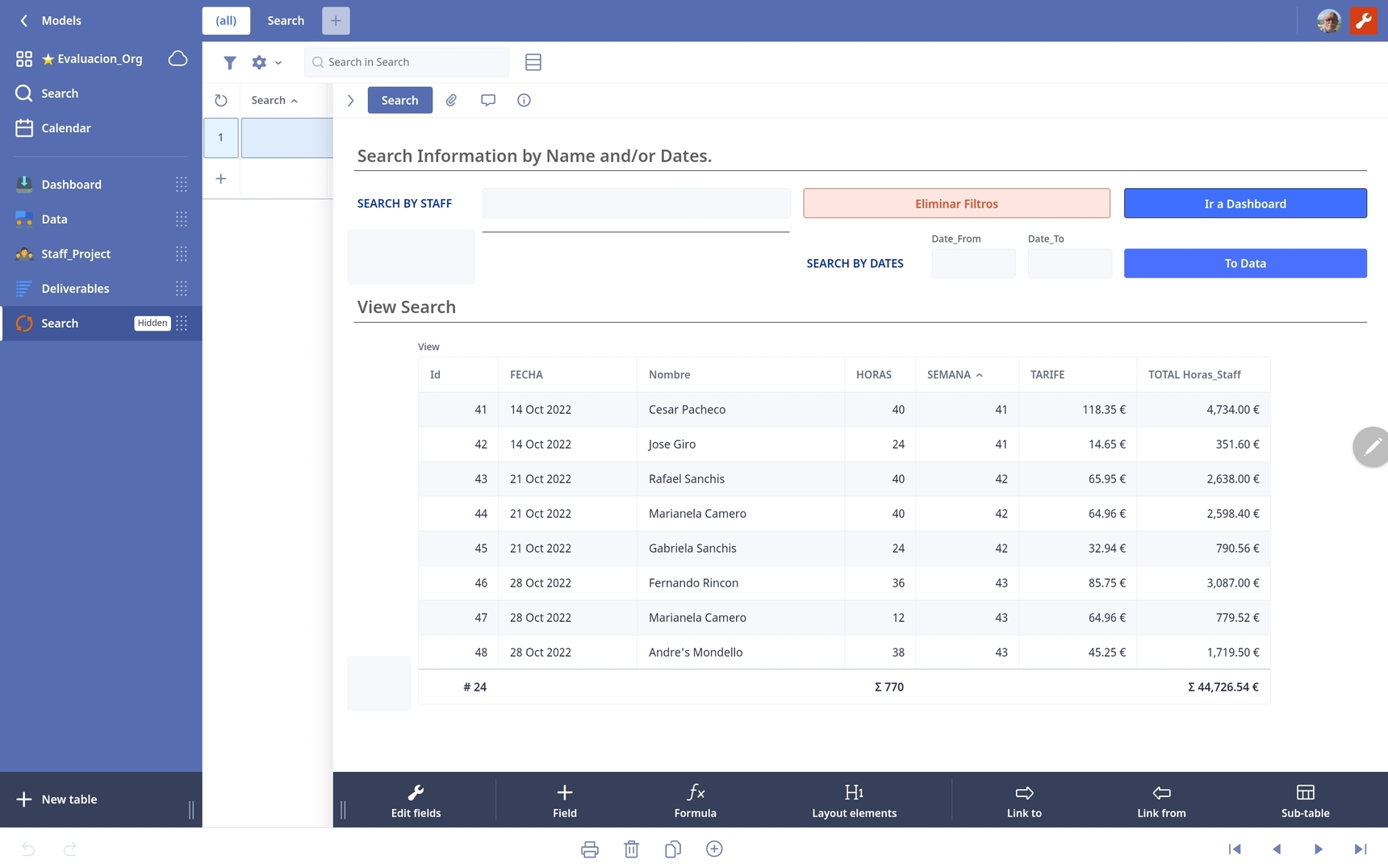0# Need Help with view table.Fred

I have the this formula and works excellent, I can search by Name and Dates at the same time. (Two search), but I will like the view table appears without information when I open the search table. Is possible.?

``````let me := this;
let myS := "";
myS := myS + if Search then "N" else "_" end;
myS := myS + if Date_From then "V" else "_" end;
myS := myS + if Date_To then "B" else "_" end;
switch myS do
case "NVB":
(select Data)[contains(Nombre, me.Search) and FECHA >= me.Date_From and FECHA <= me.Date_To]
case "NV_":
(select Data)[contains(Nombre, me.Search) and FECHA >= me.Date_From]
case "N_B":
(select Data)[contains(Nombre, me.Search) and FECHA <= me.Date_To]
case "N__":
(select Data)[contains(Nombre, me.Search)]
case "_VB":
(select Data)[FECHA >= me.Date_From and FECHA <= me.Date_To]
case "_V_":
(select Data)[FECHA >= me.Date_From]
case "__B":
(select Data)[FECHA <= me.Date_To]
case "___":
(select Data)
end``````

Copy

Copy

16replies Oldest first
• Oldest first